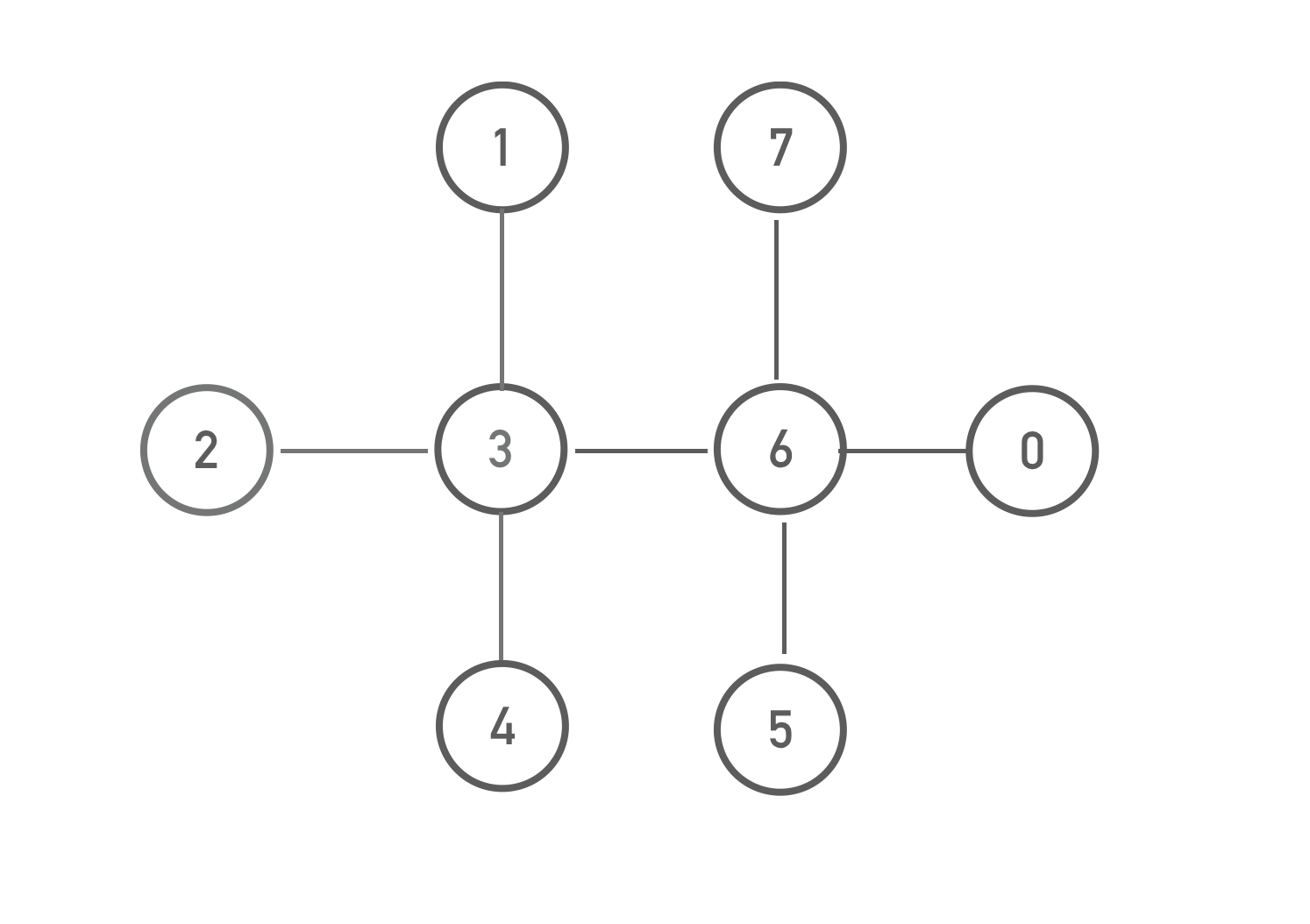# Find Critical Servers

Given an undirected graph, find out all the `vertices` when removed will make the graph disconnected.

Initially, the graph is connected.

For example given the graph below:Return `[3, 6]`. Because we can make the graph disconnected by removing either `vertex 3` or `vertex 6`.

### Input

The input consists of five arguments:

`nodeNum`: the total count of vertices in the graph, Each vertex has an unique id in the range from `0` to `nodeNum - 1` inclusive

`edges`: a list of integer pairs representing all the edges on the graph

### Output

A list of integers representing the ids of the articulation points.

### Examples

#### Input:

nodeNum = `7`

edges = `[[0,1], [0, 2], [1, 3], [2, 3], [2, 5], [5, 6], [3,4]]`